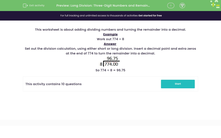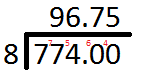# Divide Three-Digit Numbers Leaving Remainders as Decimals

In this worksheet, students will practise division of three-digit numbers by a number that does not divide exactly, leaving the remainders as decimals.Key stage:  KS 2

Curriculum topic:   Number: Addition, Subtraction, Multiplication and Division

Curriculum subtopic:   Divide to Four Digits (Long Division)

Difficulty level:#### Worksheet Overview

This activity is about dividing numbers and turning the remainder into a decimal.

Example

Work out 774 ÷ 8

Set out the division calculation, using short division.

Insert a decimal point and extra zeros at the end of 774 to turn the remainder into a decimal.

This is to show that there are no tenths, hundredths etc. so that we can continue carrying the remainder across.So 774 ÷ 8 = 96.75

Let's have a go at some questions now.

### What is EdPlace?

We're your National Curriculum aligned online education content provider helping each child succeed in English, maths and science from year 1 to GCSE. With an EdPlace account you’ll be able to track and measure progress, helping each child achieve their best. We build confidence and attainment by personalising each child’s learning at a level that suits them.

Get started